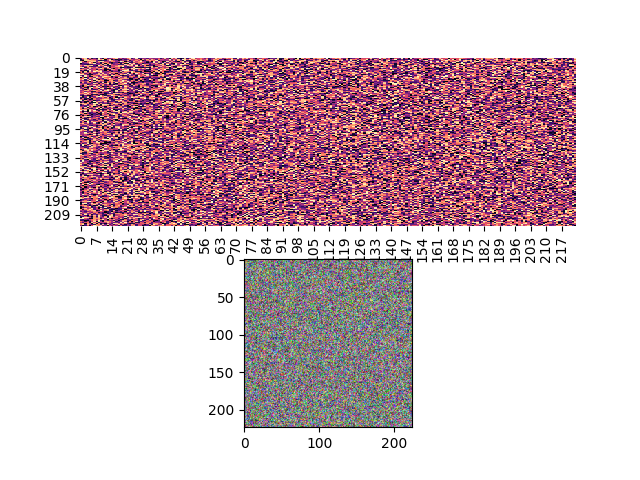# Matplotlib imshow and Seaborn heatmap inconsistency

I have two images, `im1 = np.random.rand(224,224,3) `, i.e. a colored image, and `im2 = np.random.rand(224,224)`, i.e. a heatmap of size 224x224. However, when I plot `im1` using `plt.imshow` and `im2` using `sns.heatmap`, the figures that come out seem to be of dramatically different scales. I’m a bit confused as both images are of 224x224, how can they be different in scales?

It would be a bit clearer to understand what you mean with a picture, or some sample code.

@QuLogic Here’s a code snippet

``````heatmap = np.random.rand(224,224)
image = np.random.rand(224,224,3)
fig,axs = plt.subplots(2)
ax = sns.heatmap(heatmap, cmap='magma', cbar=False, ax=axs)
axs.imshow(image)
fig.savefig('p1.png')
``````

And here’s what one would get from the code aboveBoth images are of size 224x224, why the plot shows they are of such different scales?

`Axes.imshow` sets `aspect='equal'` by default: matplotlib.axes.Axes.imshow — Matplotlib 3.6.3 documentation

`seaborn.heatmap` sets `square=False` by default: seaborn.heatmap — seaborn 0.12.2 documentation

2 Likes